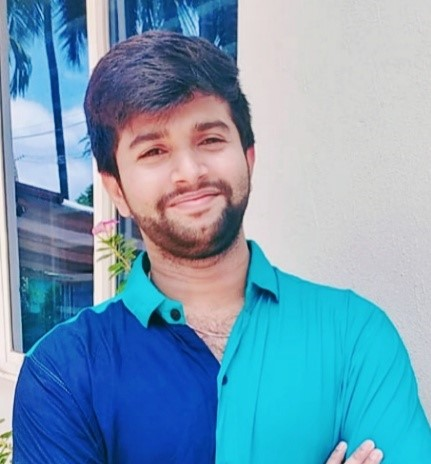This article was published as a part of the Data Science Blogathon

OpenCV is a library used for computer vision applications. With help of OpenCV, we can build an enormous number of applications that work better in real-time. Mainly it is used for image and video processing.

Along with OpenCV, we are going to use the MediaPipe library.

## MediaPipe

MediaPipe is a framework mainly used for building audio, video, or any time series data. With the help of the MediaPipe framework, we can build very impressive pipelines for different media processing functions.

Some of the major applications of MediaPipe.

• Multi-hand Tracking
• Face Detection
• Object Detection and Tracking
• Objectron: 3D Object Detection and Tracking
• AutoFlip: Automatic video cropping pipeline etc.

## Hand Landmark Model

Basically, the MediaPipe uses a single-shot palm detection model and once that is done it performs precise key point localization of 21 3D palm coordinates in the detected hand region.

The MediaPipe pipeline utilizes multiple models like, a palm detection model that returns an oriented hand bounding box from the full image. The cropped image region is fed to a hand landmark model defined by the palm detector and returns high-fidelity 3D hand key points.

Now let us implement the Hand tracking model.

Install the required modules

–> pip install opencv-python

–> pip install mediapipe

First, let us check for the working of the webcam.

```import cv2
import time
cap = cv2.VideoCapture(0)
pTime = 0
while True:
imgRGB = cv2.cvtColor(img, cv2.COLOR_BGR2RGB)
cTime = time.time()
fps = 1 / (cTime - pTime)
pTime = cTime
cv2.putText(img, f'FPS:{int(fps)}', (20, 70), cv2.FONT_HERSHEY_SIMPLEX, 1, (0, 255, 0), 2)
cv2.imshow("Test", img)```
`    cv2.waitKey(1))`

The above code will pop up a window if any webcam is connected to your PC and also shows the frames per second (fps) on the top left corner of the output window.

Now let us start the implementation. Import the required modules and initialize required variables.

```import cv2
import mediapipe as mp
import time```
```cap = cv2.VideoCapture(0)

mpHands = mp.solutions.hands
hands = mpHands.Hands(static_image_mode=False,
max_num_hands=2,
min_detection_confidence=0.5,
min_tracking_confidence=0.5)
mpDraw = mp.solutions.drawing_utils

pTime = 0
cTime = 0```

In the above piece of code, we declare an object called “hands” from mp.solutions.hand to detect the hands, in default, if you look inside the class “Hands()“, the number of hands to detect is set to 2, minimum detection confidence is set to 0.5 and the minimum tracking confidence is set to 0.5. And we will use mpDraw to draw the key points.

Now let’s write a while loop to execute our code.

```while True:
imgRGB = cv2.cvtColor(img, cv2.COLOR_BGR2RGB)
results = hands.process(imgRGB)
#print(results.multi_hand_landmarks)
if results.multi_hand_landmarks:
for handLms in results.multi_hand_landmarks:
for id, lm in enumerate(handLms.landmark):
#print(id,lm)
h, w, c = img.shape
cx, cy = int(lm.x *w), int(lm.y*h)
#if id ==0:
cv2.circle(img, (cx,cy), 3, (255,0,255), cv2.FILLED)

mpDraw.draw_landmarks(img, handLms, mpHands.HAND_CONNECTIONS)

cTime = time.time()
fps = 1/(cTime-pTime)
pTime = cTime

cv2.putText(img,str(int(fps)), (10,70), cv2.FONT_HERSHEY_PLAIN, 3, (255,0,255), 3)

cv2.imshow("Image", img)
cv2.waitKey(1)```

Here in the above code, we read the frames from the webcam and convert the image to RGB. Then we detect hands in the frame with the help of “hands.process()”  function. Once the hands get detected we will locate the key points and then we highlight the dots in the keypoints using cv2.circle, and connect the key points using mpDraw.draw_landmarks.

The entire code is given below

```import cv2
import mediapipe as mp
import time```
```cap = cv2.VideoCapture(0)

mpHands = mp.solutions.hands
hands = mpHands.Hands(static_image_mode=False,
max_num_hands=2,
min_detection_confidence=0.5,
min_tracking_confidence=0.5)
mpDraw = mp.solutions.drawing_utils

pTime = 0
cTime = 0

while True:
imgRGB = cv2.cvtColor(img, cv2.COLOR_BGR2RGB)
results = hands.process(imgRGB)
#print(results.multi_hand_landmarks)

if results.multi_hand_landmarks:
for handLms in results.multi_hand_landmarks:
for id, lm in enumerate(handLms.landmark):
#print(id,lm)
h, w, c = img.shape
cx, cy = int(lm.x *w), int(lm.y*h)
#if id ==0:
cv2.circle(img, (cx,cy), 3, (255,0,255), cv2.FILLED)

mpDraw.draw_landmarks(img, handLms, mpHands.HAND_CONNECTIONS)

cTime = time.time()
fps = 1/(cTime-pTime)
pTime = cTime

cv2.putText(img,str(int(fps)), (10,70), cv2.FONT_HERSHEY_PLAIN, 3, (255,0,255), 3)

cv2.imshow("Image", img)
cv2.waitKey(1)```

The output is:

Now let us create a hand tracking module, so that we can use it in other projects.

Create a new python file, First let us create a class called handDetector with two member functions in it, named findHands and findPosition.

The function findHands will accept an RGB image and detects the hand in the frame and locate the key points and draws the landmarks, the function findPosition will give the position of the hand along with the id.

Then the main function where we initialize our module and also we write a while loop to run the model. Here you can import this setup or the module to any other further related project works.

The entire code is given below

```import cv2
import mediapipe as mp
import time```
```class handDetector():
def __init__(self, mode = False, maxHands = 2, detectionCon = 0.5, trackCon = 0.5):
self.mode = mode
self.maxHands = maxHands
self.detectionCon = detectionCon
self.trackCon = trackCon

self.mpHands = mp.solutions.hands
self.hands = self.mpHands.Hands(self.mode, self.maxHands, self.detectionCon, self.trackCon)
self.mpDraw = mp.solutions.drawing_utils

def findHands(self,img, draw = True):
imgRGB = cv2.cvtColor(img, cv2.COLOR_BGR2RGB)
self.results = self.hands.process(imgRGB)
# print(results.multi_hand_landmarks)

if self.results.multi_hand_landmarks:
for handLms in self.results.multi_hand_landmarks:
if draw:
self.mpDraw.draw_landmarks(img, handLms, self.mpHands.HAND_CONNECTIONS)
return img

def findPosition(self, img, handNo = 0, draw = True):

lmlist = []
if self.results.multi_hand_landmarks:
myHand = self.results.multi_hand_landmarks[handNo]
for id, lm in enumerate(myHand.landmark):
h, w, c = img.shape
cx, cy = int(lm.x * w), int(lm.y * h)
lmlist.append([id, cx, cy])
if draw:
cv2.circle(img, (cx, cy), 3, (255, 0, 255), cv2.FILLED)
return lmlist

def main():
pTime = 0
cTime = 0
cap = cv2.VideoCapture(0)
detector = handDetector()

while True:
img = detector.findHands(img)
lmlist = detector.findPosition(img)
if len(lmlist) != 0:
print(lmlist)

cTime = time.time()
fps = 1 / (cTime - pTime)
pTime = cTime

cv2.putText(img, str(int(fps)), (10, 70), cv2.FONT_HERSHEY_PLAIN, 3, (255, 0, 255), 3)

cv2.imshow("Image", img)
cv2.waitKey(1)

if __name__ == "__main__":
main()```

The output will be the same as shown above along with the positions of the tracked hands.

The entire code is also available here.

Reference:

Thank you.

The media shown in this article are not owned by Analytics Vidhya and are used at the Author’s discretion.•